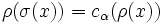# Conjugacy-separable and aperiodic implies every extensible automorphism is class-preserving

This article gives the statement and possibly, proof, of an implication relation between two group properties. That is, it states that every group satisfying the first group property (i.e., conjugacy-separable aperiodic group) must also satisfy the second group property (i.e., group in which every extensible automorphism is class-preserving)
View all group property implications | View all group property non-implications
Get more facts about conjugacy-separable aperiodic group|Get more facts about group in which every extensible automorphism is class-preserving

## Statement

Suppose$G$ is a Conjugacy-separable group (?) (i.e., any two non-conjugate elements can be realized as non-conjugate elements in some finite quotient) that is also an Aperiodic group (?): no non-identity element has finite order. Then, every Extensible automorphism (?) of$G$ is a Class-preserving automorphism (?).

## Proof

Given: A conjugacy-separable group$G$ with only finitely many primes$p$ dividing the orders of elements of$G$. An extensible automorphism$\sigma$ of$G$. Two elements$x,y$ of$G$ that are not conjugate.

To prove:$\sigma$ cannot send$x$ to$y$.

Proof:

1. There exists a normal subgroup$N$ of finite index in$G$ such that the images of$x$ and$y$ are not conjugate in$N$: This follows from the definition of conjugacy-separable.
2. Let$\overline{G} = G/N$. Then, there exists a prime$p$ such that the field of$p$ elements is sufficiently large for$\overline{G}$: This follows from fact (2).
3. The field of$p$ elements is a class-separating field for$\overline{G}$. In particular, there is a finite-dimensional linear representation$\rho_1:\overline{G} \to GL(V)$ of$\overline{G}$ over this field such that$\rho_1(\overline{x})$ and$\rho_1(\overline{y})$ are not conjugate: This follows from fact (3).
4.$\sigma$ is linearly pushforwardable over the prime field with$p$ elements, for the$p$ chosen above. In particular, if$\sigma(x) = y$, then$\rho(x)$ and$\rho(y)$ are conjugate for any representation$\rho$ over this field: Let$\rho$ be a representation of$G$ over this field. Let$V$ be the corresponding vector space and$H = V \rtimes G$ the semidirect product for the action. Since$p$ does not divide the order of any element of$G$,$V$ is the set of elements of$H$ of order dividing$p$. In particular,$V$ is characteristic in$H$, and thus, if$\sigma$ extends to an automorphism$\sigma'$ of$H$, then$\sigma'$ also restricts to an automorphism$\alpha$ of$V$. Fact (1) thus yields that$\rho \circ \sigma = c_\alpha \circ \rho$, so$\sigma$ is linearly pushforwardable over the field of$p$ elements. In particular, if$\sigma(x) = y$, then$\rho(\sigma(x)) = c_\alpha(\rho(x))$, so$\rho(y)$ is conjugate to$\rho(x)$ by$\alpha$.
5. Let$\rho_1$ be the linear representation chosen in step (3), and let$\rho = \rho_1 \circ p$ where$p:G \to G/N = \overline{G}$ is the quotient map. Then,$\rho(x)$ and$\rho(y)$ are not conjugate in the general linear group$GL(V)$. However, by step (4), we have that$\sigma$ is linearly pushforwardable, so if$\sigma(x) = y$, then$\rho(x)$ and$\rho(y)$ are conjugate. This gives a contradiction, so we cannot have$\sigma(x) = y$, and we are done.